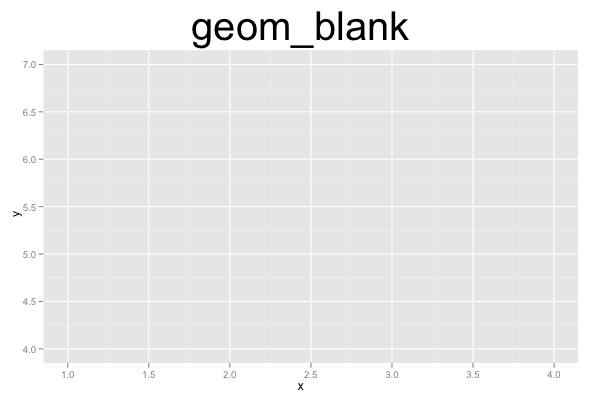## ggplot2 Quick Reference: geom_blank

A geom that draws nothing. It just creates an empty layer.

### Parameters

`geom_blank()` has no parameters.

### Example

Note that while `geom_blank()` draws nothing, ggplot2 needs a way to compute the x and y scales of the plot. Thus, we have to provide a data frame and an x and a y aesthetic somewhere. This could be as arguments to `geom_blank()`, but here we pass the information as arguments to `ggplot(...)` itself.```d=data.frame(x=c(1,2,3,4), y=c(4,5,6,7))
ggplot(data=d, mapping=aes(x=x, y=y)) +
geom_blank() +
opts(title="geom_blank", plot.title=theme_text(size=40, vjust=1.5))```